## Wednesday, February 24, 2010

### FFT (Fast Fourier Transform) of time series -- promises and pitfalls towards trading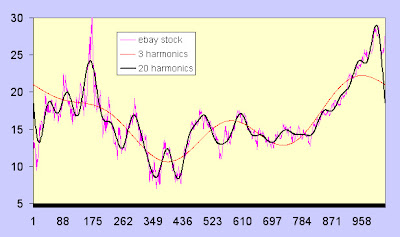Fig 1. FFT transformed time series (EBAY) reconstructed with first three and twenty harmonics, respectively.

I see quite a few traders interested in advanced signal processing techniques. It is often instructive to see why they may or may not be useful. The concept behind fourier analysis is that any periodic signal can be broken down into a taylor series or sum of suitably scaled sine and cosine waveforms (even a square wave!). The key requirement is that the signals are periodic, which means that they repeat forwards and backwards to plus and minus infinity. Anyone who deals with financial series knows they are aperiodic (meaning they do not repeat indefinitely). The FFT, or fast fourier transform is an algorithm that essentially uses convolution techniques to efficiently find the magnitude and location of the tones that make up the signal of interest. We can often play with the FFT spectrum, by adding and removing successive tones (which is akin to selectively filtering particular tones that make up the signal), in order to obtain a smoothed version of the underlying signal.

In the posted example, I showed the effect of reconstructing the transformed waveform using only the first three tones (and cutting off all others), where we see a low passed version of the signal. The second example includes the first 20 tones, which begins to match the signal more closely, but is a smoothed representation of the signal, which is often a nice representation to isolate smoothed signal component from high frequency noise. Notice the terms tones and harmonics are practically synonymous for purposes of this discussion (a harmonic is more specifically a multiple of the fundamental tone); both represent the spectral frequency components that sum up to make the total waveform. The major problem that I wanted to illustrate with this simple example (among many), is the problem of 'wraparound effects.' As I mentioned earlier, one of the requirements for properly applying a fourier transform is that the signal is periodic or repeating, since the basis functions (sines and cosines) that are convolved are infinitely repeating functions.

With that requirement, the reconstructed waveform tries its best to match the beginning and endpoints for periodic repetition. The result is severe problems at the endpoints (left and right), which are often the points we are most concerned about. So it often pays to be cautious when hearing about applications of higher level signal processing techniques. There are several other requirements and limitations to applying FFT techniques, among them: requirement of 2^n samples, fsample must be greater than or equal to twice the max bandwidth of sampled signal (nyquist criterion), limited spectral tone bin resolution; ignoring any of these issues can cause severe reconstruction errors.

## Monday, February 22, 2010

### Time Series Calendar Heat Maps Using R

I came across an interesting blog that showcased Charting time series as calendar heat maps in R . It is based upon a great algorithm created by Paul Bleicher,CMO of Humedica. I'll let you link to the other blog to see more details on the background and original source code.

I made a very small modification to allow %daily changes, rather than price values.
stock.dailychange<-100*(diff(stock.data$Adj.Close,lag=1)/y[1:length(stock.data$Adj.Close)-1])calendarHeat(stock.data$Date[1:length(stock.data$Date)-1], stock.dailychange, varname="SPY daily % changes(CL-CL)")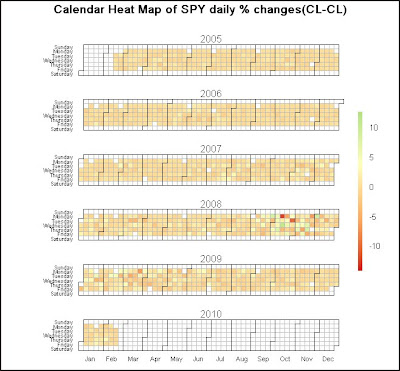Fig 1. Calendar Heat Map for SPY time series 2005-Present

What's interesting is you can see how unusual events tend to Cluster (heteroscedasticity) , and a preponderance of low change days (as would be expected in close to Gaussian distributions). Using the regions of clustering might help warn of impeding catastrophic regimes (as seen in late 08), similar to using VIX as a proxy. In addition, the 10,000 foot bird's eye view, might allow you to spot pockets of order for further evaluation.

## Saturday, February 20, 2010

### Genetic Algorithm Systematic Trading Development -- Part 3 (Python/VBA)

As mentioned in prior posts, it is not possible to use the standard Weka GUI to instantiate a Genetic Algorithm, other than for feature selection. Part of the reason is that there is no generic algorithm to instantiate a fitness function. The same flexibility that allows an infinite possible range of fitnesses also requires custom scripting. Although it is possible to write a custom class for Weka/JAVA, I chose to utilize Python for this example, along with an older VBA tool I developed for the back-end results summary. Hopefully, you'll see that there are many tools that may be utilized to prototype various systems and augment the development process.

The essential GA uses a 17 bit string length to encode the following rule:

{if ma(m) binop ma(n) then buy}

The first 8 bits are used to encode the 1st ma value. Note there are 2^n = 2^8 = 256 potential decimal values that can be used for the parameter argument. The 9th bit is a 2 bit encoded value of the > or < binary operator as discussed in prior posts. The last 8 bits are used for the 2nd moving average parameter value. A simple fitness of the net dollar return was used for this example (Note Sharpe ratio, and other fitness metrics could have been used). The input series is SPY, using the range from 1993-2005 daily to optimize.

The python script was essentially set up to run 40 generations of a population of size 20 using elitism and tournament selection. Although this is by no means optimal (it is quite small), it was set up using these values for illustrative purposes. When you watch the video, what you'll see is the initial population in binary encoded strings each time a generation is passed. In addition, the decoded moving average rule is shown for each selection change. Although the video has been truncated for brevity, you should notice that the fitness number is improving each generation. The final solution was designed to halt after a fitness did not improve over five generations. In addition, you can see the final encoded result and a plot of the fitness convergence.

Video 1. Optimization of MA parameters using Python GAFig 1. Final Fitness result output to console

In fig 1. we see that the final rule converged to {if ma(220) > ma(221)) then Buy.
In addition, the final binary string is shown along with the final fitness value.
We can decode the binary string with relative ease.
 is the 17 bit string representing the optimal fitness.
ma1 is 1st 8 bits = 11011011 = 219 decimal a +1 offset was used (so as not to have 0 day moving average) to get a resulting parameter argument of 220.
The next bit is = 1 corresponding to >
The final 8 bits represent the 221 argument by similar reasoning as the first.
So the resulting rule with parameters is:
if ma(220) > ma(221) then Buy
fitness = net$gain =$316.12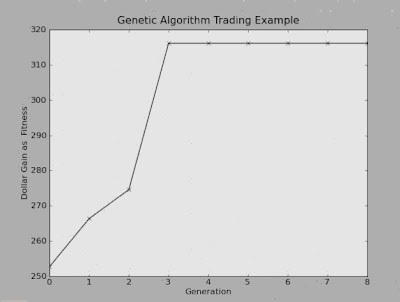fig 2. Fitness Convergence

In fig 2. We see how the fitness continued to climb over successive generations until early convergence caused a halt at the fitness value that did not change over the prior 5 generations.

In order to verify the results, we will also show how other tools may be used. In this case, I used an older VBA simulator that I wrote a few years back.

Video 2. Summary of optimized parameters using VBA/Excel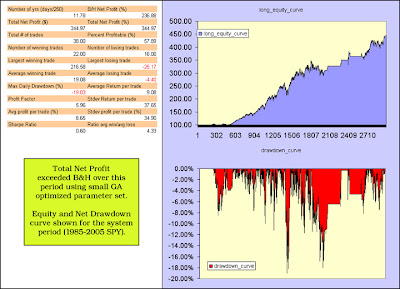Fig 3. Summary of Back Test Results

Above is a capture of the summary statistics using the back test program. Total net profit is slightly higher than the python results. This is due to the fact that the python simulation truncated the series length of the moving average data, so as to avoid zero front padded values, while the excel program did not. However, they are still in close agreement. It's often useful to use several different programs to force yourself to double check results.

Now, as an astute commenter already pointed out... this method is indeed curve fitting. What we found was the best possible pair of parameters(or at least one of the best; there are superior parameters, but I didn't run the example generation set too long) for our particular rule set we set out to investigate. Or as I mentioned in the first thread, we zeroed in on the region of the distribution curve with the most profitable candidates. Now, for those of you not familiar with curve fitting, it is not a happy concept amongst developers. In fact, it suffers from almost the same egregious problems as cherry picking examples, as I mentioned earlier on.

That being said, however, it is not done in vain. Our goal here is to quantitatively augment common development (the part where you create and verify) tools beyond mere guessing, intuition, and cherry picking. Firstly, it is possible that this particular rule set will not fare as well out of sample, which is true. However, in the same sense that we can not just take one cherry picked example for granted, we must also evaluate how things actually do perform out of sample. I say this because I've used similar techniques that looked very good, and did indeed perform very well out of sample for several periods out into the future. By honing in on the best candidates, we help to narrow down the set of candidates that are worthy of out of sample investigation. There are other additional techniques (some mentioned earlier, such as ensemble methods, different objective/fitness functions, and even different optimization criteria) that can be used to enhance this method, and in addition, verify robustness out of sample.

edit: Just for giggles, I decided to actually run the Out of Sample performance on this optimized in sample trained rule. The following chart illustrates how it performed 'out of sample' for the years 2005-today(2010).Fig 4. Out of Sample Test Performance on optimized training rule parameters.

Not all that shabby for that curve fitted simple system during the worst meltdown in recent history, eh (much easier on the gut)?

To be frank, I have run so many evaluations on simple SMA systems, that I would say that they are not the most superior parameters to optimize around. Obviously, however, it really depends on what your objective is. There are some long term studies that have shown using the fitness objective of reduced volatility as the goal is quite beneficial with this simple rule set (you can verify that this simple system had far less volatility over the down periods, than the actual market-- in and out of sample) . It is up to you to find those parameters that are worthy of optimizing further. See commentary on A Quantitative Approach to Tactical Asset Allocation for a related example.

And please continue to give your feedback on what you like or don't like and areas you want to explore.
---------------------------------------------------------------------------------
If you are new to Python and would like to order a fantastic textbook, I highly recommend the following (applications geared a bit towards science and engineering): A Primer on Scientific Programming with PythonIn addition, users who are interested in learning a bit more about VBA with a Financial Oriented slant will find great practical examples in the text: Financial Modeling, 3rd Edition## Wednesday, February 17, 2010

### Genetic Algorithm Systematic Trading Development-- Part 2

We started by discussing the goal of a genetic algorithm, which is to optimally find the candidate pool of rules that are superior to other potential rules. In our example of moving averages, we are seeking the values of parameters of the rule :
if ma(m) binop ma(n) then action.
*Note: binop is short for binary operator; in this case the binary operator is > or <.

The GA (genetic algorithm) works by encoding the rule set into a string of binary valued variables. For instance if we wanted to encode the moving average parameter
to 4 real decimal values, we could simply use a 2 bit string, where 00 = 0 decimal, 01= 1 decimal, 10 = 2 decimal and 11 = 3 decimal. We can encode up to 2^n values per bits contained in each string. If we wanted to encode 512 values, we would need a 9 bit string to encode this value (2^9=512).

Also, we can encode values other than decimal values as binary bits, for instance,
action = buy or sell, can be represented by 0 or 1. Greater or Less than (> or <) can be represented by 1 or 0, as well. In the end we will have a chromosome or total string that represents the rule we are trying to optimize. So the rule: if {ma(m) binop ma(n) then action} could be encoded by binary values, as each chromosome is represented by 4bits- 2bits- 4- bits- 2 bits, where each element of the rule string corresponds to the encoded values discussed above. Note that the encoded blocks would be comparable to genes.Fig 1. Examples of Boolean Encoding

Once we encode our rule set into a boolen representation or string, we then want to generate a population of strings to select from. Typically, we start out by assigning random values to the parameters. For instance, we may start a population of 100 strings; each string representing a set of rules with different parameters.
One string could be if ma(10)<ma(50) then buy, another might be if ma(20)>ma(200) then sell. Once a population has initially been created, we need to create diversity and additionally successfully improve fitness in the offspring over successive generations.

The concept of fitness is perhaps one of the most elegant and flexible options that makes the GA such a powerful optimizer. In the decision tree learners and Neural Network learners we discussed, there are only one or two simple goals to train on (decision tree for instance trains towards goal of reducing information entropy, neural net trains on reducing fitted variance errors). The GA can use any goal you can imagine, which gives it unlimited flexibility compared to others. You could use
total gain as a goal, or sharpe ratio, or profit factor as goals. You could even combine goals. The fitness or goal is what you are trying to optimize. Keep in mind that a genetic algorithm, like any other learner does not guarantee you will find the absolute best, it may get caught in local maxima of the fitness landscape.
However, you can get more sophisticated and add other sub methods to try to avoid this.Fig 2. Example of population of rules to be processed.

Once our initial population of parameter based rules has been created (randomly), we then want to think about how we achieve the goal of optimizing or finding the set of rule parameters that best optimizes our fitness. Note that each time we create a new population of offspring, we call this a new generation run or epoch. The first set of offspring or parents, commonly attempts to select some sample of the fittest members to be passed along to the next population. We could use a greedy method that just sorts or ranks the members and selects only from the best 50% to be passed to the next generation (known as truncation selection) or an alternate method is to use something called roulette selection. In roulette selection, we sample members of the original population based upon their normalized fitness. So if the best fitness was 20% of the value of the sum of all the finesses, we would copy over that string or rule with a 20% probability into the next generation. The same would be applied to the other fitness/string combinations. Ultimately, we end up with more of the offspring selected from the most fit candidates in the prior generation. Next, we want to assure some diversity in the offspring. Crossover operation achieves this by crossing over or swapping genes from one candidate and another. This is performed over the entire population to ensure diversity. Lastly, we use mutation to randomly select some number of string elements and flip them. It adds a bit more random diversity to the offspring, so that possibly some candidate may show up unexpectedly that has great performance (think unusual height in basketball for instance).
More bells and whistles can be added to improve performance. Tournament selection is another method that improves offspring selection by running a tournament between string candidates. The winning candidate gets passed along to the next generation.Fig 3. Selection process.

We essentially run the optimization and diversity routines through each generation, and the best candidates get passed down through to the next generation until our number of generations has run out, or we specify an early stopping criterion.

In the case of our rule set, we expect it to converge to the best set of parameters
(moving average arguments, and binary greater or equal than operator), based upon the fitness goal we assign to it.

Next. Genetic Algorithm Systematic Trading Development-- Part 3

## Monday, February 15, 2010

### Genetic Algorithm Systematic Trading Development -- Part 1

I want to start with a brief introduction to what I consider one of the most powerful learning methodologies to come out of Artificial Intelligence in the last several decades-- the Genetic Algorithm. Although it was originally developed to model evolutionary biology in the late 50s, most give credit to John Holland for his detailed contributions to the development of the field. A professor of adaptive systems at the University of Michigan, he wrote a text, titled "Adaptation in Natural and Artificial Systems" in 1975, that is considered a landmark book in the field.

Although GAs are designed to borrow from our Genetic Biology, I want to quickly describe why they are powerful with respect to trading systems development. As a trader, you might often develop systems using creative ideas borrowed from Technical Analysis books you may have read. One of the problems with earlier TA books in general, IMO, is that they often have "cherry picked" examples of parameter sets, without much explanation as to how they arrived at the parameters, nor how well they might perform against other parameters. In statistics, we are often interested in gathering many different samples to build a distribution profile of the outcomes as an estimate of the true population of all possible candidate solutions. We often look at these distributions of models to gather a quantitative deduction about whether our particular system (with the parameters we selected) has performed better than any other potential system in the universe of all possible candidate solutions.

If the system performed better than some designated percentage of the sample distribution area of 100% (often set at 1% or 5% in common literature), then we can say that the result compared to the universe of candidates is "statistically significant". Using different parameters for the same set of systematic rules will give different sample outcomes that make up that distribution. For instance, using moving average crossovers, we might end up selecting one pair of moving average values to determine entry and exit with a resulting profit of .1%, while another combination over the same period yielded 2.3%. Ultimately we want to find the set of pairs that performs the best, or at least significantly better than Buy and Hold, otherwise there's typically not much incentive to trade in and out as commission costs and other negative effects make it prohibitive. We could try to run various parameters by guessing or enumerating over the search space of potential solutions, but at a certain point, the number of combinations becomes unwieldy and is not computationally efficient. The first step might be to evaluate the parameters of our system and look for those parameters that yield statistically significant results, the next might be to compare that candidate to buy and hold or other potential system candidates using a t-test of the separate distributions.

Let's take an example of a potential set of rules to illustrate this idea. Suppose we sat down one day and decided upon a rule that said to buy if the m period moving average was greater or less than the n period moving average. First, we need to decide upon what range of values to use for the averages. If we discretize the range of values to integer values, i.e. 1 to 512 steps each, we would have 512X512x2 (where 2 represents greater or less than)= 542,288 different parameters to enumerate through (or try). Although that doesn't seem too large of a number of combinations to try with today's computational power, as we begin to make the rules more complex, the number of combinations will begin to run into the millions. It's just not feasible to try all of them, so we want to find some method to reduce the number of potential candidates, while at the same time finding the best possible results. What we are trying to do is find an 'optimal' algorithm to converge to the best solutions quickly. There are numerous algorithms employed in the field of machine learning, under the category of optimization algorithms that exist to achieve this goal. The genetic algorithm is one such optimization algorithm that borrows directly from our own evolutionary genetic system to find the best potential candidate, without having to literally try out every single possible combination.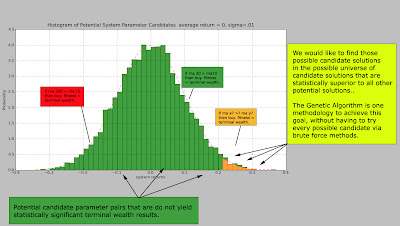Fig1. Example of searching for statistically superior parameters.

Above, we see an example distribution of possible candidate solutions in the population of potential parameter pairs with the x-axis representing binned ranges of potential gain for the system, and y representing the frequency of parameter pair outcomes corresponding to that gain. Our Genetic Algorithm will help us to find those solutions that are statistically significant compared to potential solutions.

Next: Genetic Algorithm Systematic Trading Development -- Part 2

## Thursday, February 11, 2010

### Artificial Immune Systems and Financial Applications?

One of the buzzwords that seems to be common these days is AIS or Artificial Immune Systems. It is a biologically inspired classification type system that essentially tries to replicate some of our own natural immune system algorithms. Our bodies have various defense mechanisms for recognizing foreign invaders. One such defense mechanism is utilizing pathogen-associated molecular patterns built into our genome so that we can identify foreign pathogens and respond to them. This concept of being able to distinguish between self and non-self is essentially one of the main themes that is borrowed from our natural biological defense.

The idea is that we can learn to recognize objects that are not normal and respond to them. A good example where this has been put to use is in SPAM detection. We want to be able to recognize good (known) mail from the (unknown) bad, and utilize AIS to avoid parasitic SPAM.

The most common AIS system that has been adopted is the Negative Selection Algorithm. It essentially works by training a classifier to generate negative examples randomly and if the negative example (imagine a coordinate on a 2D grid) happens to be located (using some distance metric, such as euclidean or mahanalobis distance) near enough to a known good example, then it is rejected. Essentially, we are randomly generating negative examples for the classifier to identify.Fig 1. Basic concept of AIS type classification

Although it has been used in some cases for applications like credit detection, one of the things you'll notice in AI based algorithms is that there are many other algorithms that might do the same job or better. In the case of trading systems, we might want to identify abnormal time series behavior and make a decision based upon this information, however, it is might be simpler to use statistical control methods to better ascertain the information.

In conclusion, although the idea sounds promising, I haven't seen many practical superior examples that would benefit a trading system that cannot be employed by existing AI algorithms.

artificial immmune systems

There is a wealth of good reading in the linked site, as well as a Weka plugin that can be used to access this algorithm for readers following the Weka tutorials.

## Wednesday, February 10, 2010

### Using J48 Decision Tree Classifier to Dynamically Allocate Next Day Position in Stocks or Bonds

The prior introduction using a simple model to determine next weeks change based on the S&P 500 index and VIX did not look very promising, although hopefully it served to familiarize yourself with how classification is used in augmenting trading decisions. Wouldn't it be nice if we had something that performed a little better?

Well, let's look at an application of using a decision tree type classification in order to predict whether to invest in stocks or bonds one day ahead of time.
We will use a very simple input model stimulus in order to arrive at a decision.
The following will be used as input attributes.
1) VIX 1 day change
2) TLT 1 day change
3) SPY 1 day change
4) VIX 5 day momentum
5) TLT 5 day momentum
6) SPY 5 day momentum

The VIX is used as a volatility proxy to measure fear, which leads (presumably) to flights to safer instruments (bonds).

The TLT is the iShares Barclays 20+ Year Treas Bond ETF used to track treasury bonds with an average duration of 20 years.

The SPY is an ETF that tracks the general market index: S&P500.

The remaining 5 day momentum attributes are simply nominal attributes of UP or DN used to generally ascertain the momentum of the index over the last 5 days. In addition to the input attributes, we append one output attribute which is the superior instrument to invest in the following day-- SPY or TLT (stocks or bonds). This is what we are trying to predict and decide upon. The training and testing data sample is from the period 7/31/2002 up until present.

By entering the information into Weka (via .csv, see prior tutorials), we will choose the J.48 decision tree learner and use 90%/10% training/test split in order to develop a model tree that will predict which class of instrument to invest in based upon the prior days input stimulus.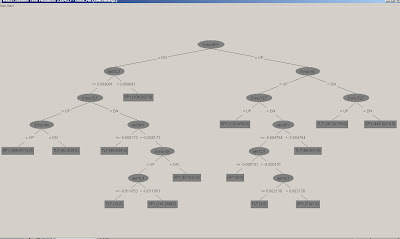Fig 1. Resulting Model Decision Tree

The decision tree can be read from the top down as making a decision based upon certain conditions. I.e. If we traverse the far left branch for example, it would give us the following rule:
IF 5 day SPY momentum is DN and 1 day TLT change is <=.91% and 5 day TLT momentum is UP and 5 day VIX momentum is UP then
invest the next day in SPY.

We can traverse each branch similarly to obtain an all encompassing set of rules to make a decision on what to invest in the following day.
Although the tree looks a bit daunting, if you can program the rule set into your favorite language, it is a simple matter for the algorithm to take that model and process it forward.

Finally, we want to see if the prediction scheme was any better or worse than guessing.Fig 2. 90/10 split train/validation results of J.48 Model Tree

The results are pretty good. Using a very simple and intuitive model, we were able to select the better instrument to buy with a 59% success rate on the 10% out of sample validation set. The same type of methodology can be used to select between trading systems with a little ingenuity.Fig 3. Equity Curve comparison of Learner System to investment classes on out of sample data

Finally, we take a look at the equity curve of investing in
1) The results of the classifier system we modeled
2) Investing in SPY or TLT alone (Stocks or Bonds)
3) Investing half in each

Notice the terminal wealth results from our system only slightly beat
all of the other systems. It's a good example of how you might have a good hit rate and only moderate improvement in net results, since hit rate does not account for magnitude. In addition, the costs associated with commission and slippage from trading many times in an out would likely overcome the systematic edge. Later on as we discuss Genetic Algorithms, we will see there are many other ways to optimize.

As always, please do your own due diligence and thoroughly verify any results you may use to make decisions in your own trading.

## Monday, February 8, 2010

### Classification for stock directional prediction

The neural network tutorial focused on a type of method known as regression. The other common method utilized in machine learning is called classification. The two approaches are somewhat similar in that they identify the best possible curve to learn from a set of data. The difference lies in how they use the curve to learn from the data. In the case of regression, we are often minimizing the distance between each exemplar and the average, whereas in classification, we are trying to discriminate between separate classes by region.

Although the following example is if anything an example of market efficiency (i.e. not much edge in terms of prediction), it serves to illustrate the basic idea of classification with application towards market prediction.Fig 1. S&P 500 weekly change vs. VIX

In the figure above, we see a common scatterplot depiction of the S&P 500 weekly return vs the VIX (which is a proxy for volatility). One common observation utilizing regression shows that the S&P 500 is negatively correlated to the VIX. Or qualitatively, large positive changes in the VIX imply negative changes in the S&P index; this is one reason it's often known as the fear index (since a rise in the VIX is associated with negative returns in the S&P 500). If we were to run a regression, we would quantitatively describe this correlation by the R^2 value of the slope, which as can be seen here visually, is a negative value.

But the regression observation says nothing about prediction into the future, it only says that there is a negative relationship between the two values at any given sample instant (in this case, weekly samples). One way to set up the prediction problem for illustration would be to use the current changes in both the S&P 500 index as well as the VIX to predict the next weeks change using a classification method.

The plot shows both UP and DN changes one week later, depicted by green and red labels. Notice that the outcome of the prediction here is nominal and not numerical, which is another common distinguishing feature between classification and regression schemes. Common methods used to deploy classification schemes are learning trees, support vector machines, and most of the tools that are also used in regression. Ideally if the classification scheme was able to discriminate classes well, it would separate classes by a curve or some type of function that would isolate both in sample as well as out of sample classes with a good separation.

Unfortunately, when data is very random, it is not able to separate classes very well.Fig 2. Plot of classification values for S&P 500 UP and DN against VIX

We can see that there is so much overlap in the UP and DN regions that it would be hard to find a curve that would classify the regions with good separation.

We use a common learning decision tree scheme called J.48 to attempt to predict out of sample results for the classifier.Fig 3. Out of sample classification results

Using the 66% In sample (training) scheme as in the NN example, we see that the predictive learner had a 53% success rate out of sample. If we compare this result to a simple naive learner (often used as a benchmark) using the last result as the prediction, we get identical results. The upshot is that using the information we have, the markets have proved efficient against this simple prediction method.

The classification concept may be extended to other applications (such as regime detection, system selection, artificial immune systems, or using multivariate input attributes) with some creativity, but the goal here was to give a simple introduction to the concept as it is the one of the most important learning concepts in machine learning. Classification may also employ supervised or unsupervised methods-- in this case it was using supervised learning (training by examples).

## Sunday, February 7, 2010

### Practical Implementation of Neural Network based time series (stock) prediction -PART 5

Following is an example of what it looks like to predict an actual univariate price series. The period of the signal that was sampled was already in stationary form, so not much massaging was needed other than normalization (described earlier).

What's important to notice when you see these kinds of neural network predictions (particularly in marketing snapshots for software vendors or trading book examples) is that they look fantastic out of sample from a bird's eye view. Unfortunately, the devil is always in the details. If you zoom way in, the predictions are not as accurate as the larger picture portrays. A more accurate method to asses how well the prediction performed is to look at the percentage change of each predicted value. We can simply compare the sign of the actual percentage change to the predicted change. In this case, the out of sample test results had a 43% hit rate, which is worse than a naive predictor would predict. The good news is you can flip those results, and just predict the opposite direction to get a 57% hit rate. However, you always have to be careful to do due diligence to verify the robustness of these types of predictions over many conditions. Another thing to be careful about is that hit rate only gives you number of correct predictions, but tells you nothing about the magnitude of the predictions, which are important to have a positive net expectation. The type of result you see here, however, is common for predicting specific univariate time series data values.Fig 1. Stock Prediction with out of sample region highlighted

You now have a practical example to get you started with building your own prediction system with free tools (except excel, which you likely have), and some ideas and methods to build your own prediction system. Any professional software you purchase will not differ much other than using different attributes to train on or modifying the internal architecture of the neural network. I have not shown more detailed examples on advanced techniques, but might incorporate some later if there is demand.

## Thursday, February 4, 2010

### Practical Implementation of Neural Network based time series (stock) prediction -PART 4

Consider this an introduction to how we need to pre-process the data.
I mentioned earlier that a financial time series is typically a unit root or non-stationary signal, what this means is that if you sample statistical properties over time, they will obviously change.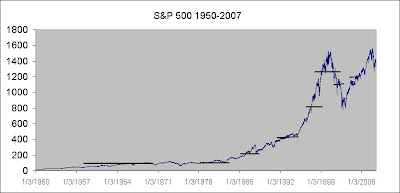Fig 1. S&P 500 non-stationary signal

You can see that as we sample the average at various points it is constantly changing. Another property of a unit root time series is that it is continuously growing (or exploding). We need to somehow transform the time series back into a stationary signal, so that the Neural Net can process and learn it. Not only is it necessary for the Neural Net to see similar if not repeating data over and over, but any values beyond the internal squashing function will get saturated at the rails of the processing elements.

One of the things that you'll notice for many long term financial time series is that they grow exponentially, so a good candidate fit might be an exponential equation. However, since we will be using decomposition detrending, I prefer to use a line fit. In order to accomplish this, we can take the log of the data and later reverse the operation for post processing. Taking the log of exponential data also transforms the exponential regression to a linear one that we can use linear regression on and subtract the time series to get some stationarity .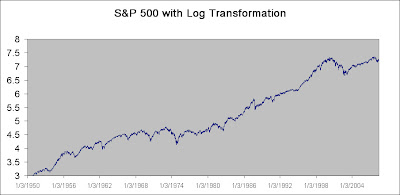Fig 2. Log Transformed Time Series

Also, notice that we will be predicting the next day, so we can simply use linear regression parameters updated daily to predict the next day.

If we have a sufficient amount of data, we should see that the parameters settle to a stable limit, much as a coin toss converges to an asymptotic limit. If the parameters settle, we have some confidence that they will not change much from one sample prediction to the next.fig 3. Dynamic Slope Settling of Linear Prediction Parameters

Notice that the parameters have settled to a pretty stable value over the training period, implying that we don't expect them to change too wildly from the true value on the next predicted estimate.

After we subtract the line regression from the log transformed signal we get our detrended and stationary signal.fig 4. De-trended Log transformed signal

Notice it appears much more stationary than the original time series. However, because the Neural Network does not get to see a lot of repetitive high frequency information over the time window, I will detrend once more with a faster smoothed representation. First we will use a 100 period moving average as the new intermediate trend, then subtract a 25 period moving average to get the 2nd detrended series.Fig 5. Second de-trended series.

Notice that even this small sample shows a much better signal for the Neural Network to learn subtle patterns in the time series, and that stationarity property is very tame.FIg 6. Reconstructed prediction Out of Sample

The figure above shows an example of a stock series that has been decomposed and smoothed then recomposed with a 100 and 25 period moving average and the out of sample period. There is a very good correlation between predicted and actual smoothed estimates. Such a system might be utilized in a moving average crossover prediction to gain a 1 day advantage in estimating momentum. There are some very small discrepancies in predicted vs actual values, however, I believe it is due to one small problem I've had with Weka. The output of Weka only outputs 3 digits numerical precision. On the nabble forum they have mentioned a newer option in Subversion, but I haven't had a chance to play with it yet.

## Monday, February 1, 2010

### Practical Implementation of Neural Network based Time Series (Stock) Prediction - PART 3

Ok, now that we have seen how well the perfect sine wave signal was learned, let's turn it up a notch and see how well the complex sine wave was learned.Fig 1. Summary of Actual Vs. Predicted out of sample complex sine waveform

Uh Oh. What happened, the out of sample data does not look quite as good. But, let's take a look at the summary statistics.Fig 2. Weka Summary for Actual vs Predicted OOS complex sine waveform

We see that the rmse went way up from 0 to about .92, even though the correlation coefficient is still pretty good looking. What's happening is that even though the signal is still perfectly deterministic, the NN needs more training data or more work on the architecture to approximate the new function properly.

Lastly, let's add some random noise to the signal.fig 3. complex sin with noise added.

And let's try to train on the random signal.fig 4. Actual vs prediction complex sin with noise added

We see that the predictions are starting to look downright bad.
The rmse went to .3, but it can be a bit misleading as the signal magnitude of the predicted waveform has dropped considerably. More importantly the correlation coefficient dropped from .9 down to .3.fig 5. Weka summary of Results.

Although the rmse doesn't look too bad, the correlation coefficient dropped from .9 all the way to .3 and relative error jumped from 15% to 97%.

Conclusion, the more noisy or high frequency the signal we train, the worse the results. Let's try to understand this from a different perspective.

Let's think about why the first simple complex predictions were so nice.
What does a neural network really do? You might have heard that it is a universal function approximator. This is essentially true. Just as a line fit, y=mx+b is a universal linear function estimate, a neural network thrives on learning any non-linear unknown general function. But, let's have a look at the scatterplot of only the original sine vs it's previous lagged value.fig 6. Scatterplot of perfect sine vs. lagged one value and time series plot.

What we notice is that when we lag the sine against itself, we see a nice deterministic pattern as we expect. This pattern is also sometimes called a lissajou pattern. But, what happens when we try to predict a value from only the previous lagged value? There are two possible outputs, pt A and pt B. If you recall way back in algebra, a function is a mapping of a set of point(s) in a range to one and only one unique output, but here we see there are two. Therefore, even if the model was perfect, it could never properly predict the next value as there are two possible outcomes; it's about as good as a coin toss. So the actual predict result would be the average of the two possible output states. But, remember we added lagged values as inputs to be trained on. Well, what happens when we do a scatterplot of the perfect sine against the prior two lagged values?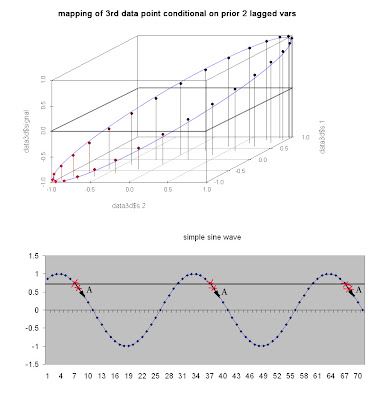fig 7. 3D Scatterplot of perfect sine wave against two lagged values and ts plot.

What we see is that by conditioning the function on the prior two lagged value pair, there is only one and only one unique corresponding output point! There is no more ambiguity, therefore there exists a perfect function that can fit this conditional prediction. This is why the first perfect sine with embedded variables had such a perfect fit on the neural network regression. It is another way to think about how a neural network learns patterns and why using embedded dimensions or lagged variables to train on is useful.

What happens though when we corrupt the sin with noise?
Here is the scatterplot.fig8. Noisy Sine Scatterplot against lagged values.

Look at all the possible ambiguous outputs each prior input predicts! It's no wonder the poor neural network has a hard time learning. It will either give some average output, or depending on the embedded dimension structure (lagged values), a very different prediction than we would expect.

In conclusion, I hope I've given you some food for thought about what a neural net likes and how it learns well.

It may need more than one lagged dimension to learn well and it does NOT like noisy inputs! This is a problem I have found with a lot of the literature that uses neural networks to predict and gives it a bad rap. They summarize using metrics like hit rate as an objective function. Yet, this is like trying to track a coin toss, it's just not always the most useful objective.

I want you to also think about it another way, as it might apply to stock prediction. Look at the following signal.fig 9. Momentum tracking with smooth sine

Take a look at what we are doing by tracking the 'smoothed' version of the sine.
We are simply tracking the momentum-- up or down (and possibly sideways)-- that's it. Or another way to think about it is we are tracking the trend, but not each little wiggle. We can also see that there is strong serial or auto-correlation in the momentum, unlike a high frequency raw time series.

By using a 'smoothed' version of a signal, we can focus on tracking the signal and not the noise. So things like hit rate are not that important. What's important is that we captured most of the meat of the trend. A secondary benefit is that we do not get bucked around and churned like a bronco as much. In communications, we use something called a phase locked loop to track clock signals embedded in time domain noise (jitter), here we are focusing on tracking the financial 'signal' embedded in the noise and not so much on each little fluctuation. It is true there will be residual fluctuations, but these drawdowns can be monitored through something like a statistical control chart, while allowing the neural net to focus on and track the signal while not getting bogged down in trying to track noise, which can be counterproductive.

Another way to think about this issue is as follows. If you are familiar with econometrics, there are no shortage of models that try to predict all of the sharp turns and high frequency components (AR, ARMA family, etc.). Normally they will tell you that if the residuals still have some serial correlation, that you have not modeled it well and it needs additional fine tuning. That is all great if you are trying to perfectly back fit a model (deductively), but it works pretty bad out of sample (inductively), because you are essentially over-fitting the model. One of the very interesting successful concepts that has come out of machine learning in recent years, is the idea of ensemble averaging methods. There are several tools like bagging, boosting, stacking, and committee voting that try to take an average prediction rather than a precise one. Predicting the averages has found much success, including the well known NETFLIX prize, where they stack learners.

If this is starting to sound foreign to you, just think about the point of this post, which is to try to smooth the signal and follow the average, rather than predict the high frequency fluctuations.

NEXT. Part 4. The Stock Prediction example.